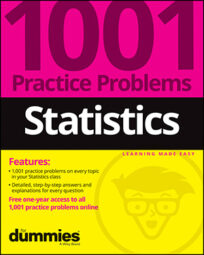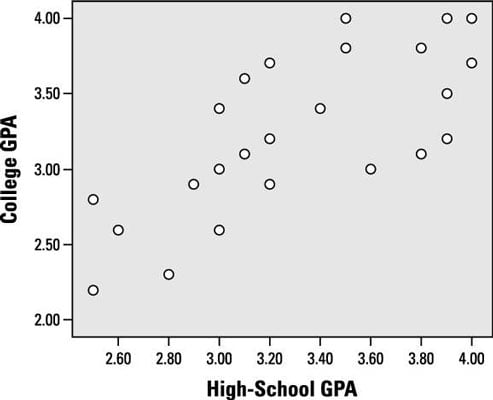##### Statistics: 1001 Practice Problems For Dummies (+ Free Online Practice)Correlation is more than just a number; it's a way of describing relationships in a universal way. Correlation applies to quantitative variables (like age and height), even though the "street definition" of correlation relates any variables (like gender and voting pattern).

This scatter plot represents the high-school and freshman college GPAs of 24 students.Credit: Illustration by Ryan Sneed

## Sample questions

1. How would you describe the linear relationship between high-school GPA and college GPA?

A. strong

B. weak

C. positive

D. negative

E. Choices (A) and (C)

Answer: E. Choices (A) and (C) (strong; positive)

This scatter plot displays a strong, positive linear relationship (r = 0.77) between high-school and college GPA.

2. If these two quantitative variables had a correlation of 1, how would the scatter plot be different?

Answer: The points would all lie on a perfectly straight line sloping upward.

With a correlation of 1.0, all the points would lie perfectly on a straight line instead of just clustering around it. The line would slope upward from left to right.

3. If these two quantitative variables had a correlation of –1, what would the scatter plot look like?

A. The points would all lie on a straight line.

B. All the points would have to be between –1 and 0.

C. All the points would slope downward from left to right.

D. Choices (A) and (C)

E. Choices (A), (B), and (C)

Answer: D. Choices (A) and (C) (The points would all lie on a straight line; all the points would slope downward from left to right.)

The scatter plot of two variables with a correlation of –1.0 would have all the points lying perfectly on a line sloping downward from left to right.

If you need more practice on this and other topics from your statistics course, visit 1,001 Statistics Practice Problems For Dummies to purchase online access to 1,001 statistics practice problems! We can help you track your performance, see where you need to study, and create customized problem sets to master your stats skills.